§ 3 Hypergeometric functions

1.     Gaussian hypergeometric series *

[ Gaussian hypergeometric series and its analytical development ]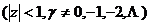called a Gaussian hypergeometric series, it is a hypergeometric differential equation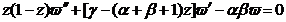, and within single-value parsing .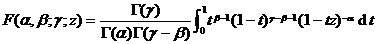is an analytical expansion of a Gaussian hypergeometric series into the removed plane, also known as a hypergeometric function .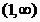[ Integral expressions for hypergeometric functions ]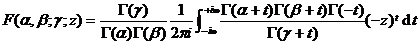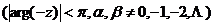The integration route should be selected so that the pole of the function is on the left side of the route, and the pole of the function is on the right side of the route .This integral is called the Barnes integral representation .

[ Recursive formula and related formulas ]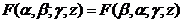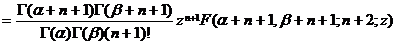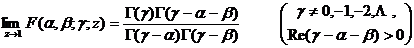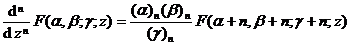[ Conversion formula ]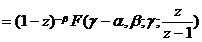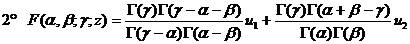in the formula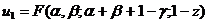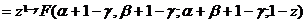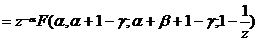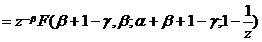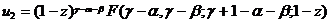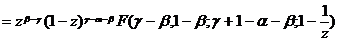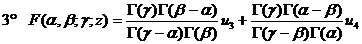in the formula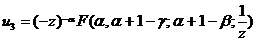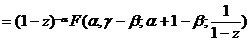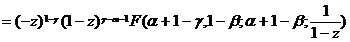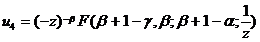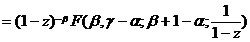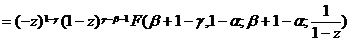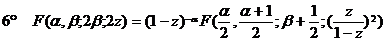[ asymptotic expression ]fixed,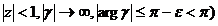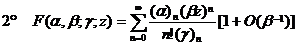fixed,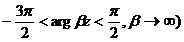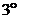At that time , the transformation formula is an asymptotic expression of .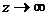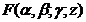2.      Kummer function (confluent hypergeometric function)

[ Kummer function and its integral expression ]   series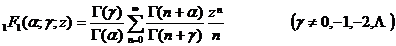is called the Kummer function (or confluent hypergeometric function), which is an integral function of the variables and parameters , respectively . It satisfies the Kummer differential equation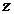Its integral expression has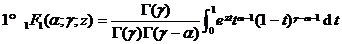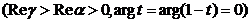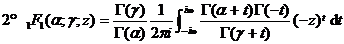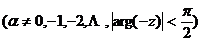The selection of the integral route should make the pole of the function on the left side of the integral route and the pole on the right side of the integral route . This formula is called the Barnes integral representation .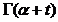[ Recursive formula and related formulas ]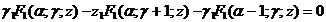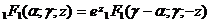(Kummer transform)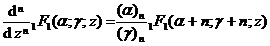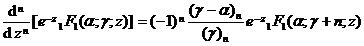[ asymptotic expression ]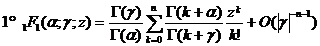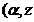Have bound,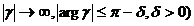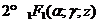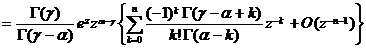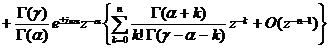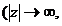In the formula, the sign is selected as follows: the positive sign is taken at that time,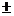Take a negative sign at that time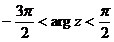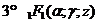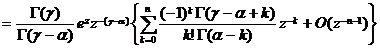* The general form of a hypergeometric series is

The formula is called the numerator parameter and the denominator parameter . The convergence is as follows:

At that time, it converges for all finite .

At that time , everything that satisfies is convergent .

At that time , divergent for all .